#### Howdy, Stranger!

It looks like you're new here. If you want to get involved, click one of these buttons!

Options

# Lecture 37 - Chapter 3: Presentations of Categories

edited February 2020

Last time we saw that for any graph $$G$$ there is a free category on that graph, whose objects are nodes of $$G$$ and whose morphisms are paths in $$G$$.

We can get more categories using another trick: we can add equations between paths in the graph, and still get a category! We are only allowed to equate two paths $$f$$ and $$g$$ when they are parallel, meaning $$f : x \to y$$ and $$g: x \to y$$ for some objects $$x$$ and $$y$$.

For example, last time we saw a category coming from this graph: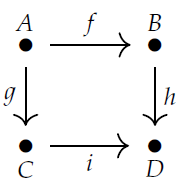This category is called the free category on a square. In this category there are two paths from $$A$$ to $$D$$, namely $$h \circ f$$ and $$i \circ g$$. They're not equal!

But we can make up a new, smaller category where we artificially decree that $$h \circ f = i \circ g$$. This category is famous: it's called the commutative square since you can either go down and then go right to get from $$A$$ to $$D$$, or go right and then go down, and we are counting these as the same morphism.

Both these categories are important. Imagine living on a city block with one-way roads. You live at $$A$$ and you are trying to visit your friend at $$D$$. For some purposes it may matter which route you take. Then you should use the free category on the square. But for some other purposes it may not matter - you may not care about how you're going from $$A$$ to $$D$$, just whether you can do it. Then you should use the commutative square!

Remember from Lecture 35 that a preorder can be viewed as a category with at most one morphism from any object to any object. The commutative square is a nice example: in the commutative square, there's at most one morphism from any object $$A,B,C,D$$ to any other. We use preorders when we don't care how we go from one object to another, just whether you can.

Indeed, we can always take any category $$\mathcal{C}$$ and artificially throw in so many equations that we collapse it down to a preorder! This was the topic of Puzzle 103. To do this, we simply decree that decree $$f = g$$ for all pairs of morphisms $$f, g : x \to y$$, for all pairs of objects $$x$$ and $$y$$. The result is called the preorder reflection of $$\mathcal{C}$$.

Fong and Spivak discuss this in Section 3.2.3, and they make a good point: the free category on a graph is very different from a preorder. The free category on a graph has the fewest possible equations between parallel morphisms, while a preorder has the most possible.

Anyway, our trick of building a category by first taking the free category on a graph and then imposing some equations between parallel morphisms is called presenting a category. The graph, together with the equations, is called a presentation of the category.

If we use a finite graph and finitely many equations, we get a finitely presented category. These will be important for databases.

For example, consider this graph:It has 3 nodes: Employee, Department and String. It has 5 edges: WorksIn, Secretary, DepartmentName, FirstName and Manager. If you think about it, you'll see what's going on. Every employee has a first name, which is a string of characters, and that's why we have an edge FirstName from Employee to String. In the free category on our graph, this edge gives a morphism

$$\textrm{FirstName} : \textrm{Employee} \to \textrm{String}.$$ Similarly for the other edges. Every department has a secretary, which is an employee, and so on. We also get composite morphisms like

$$\textrm{FirstName} \circ \textrm{Manager} \circ \textrm{Manager} : \textrm{Employee} \to \textrm{String}$$ This says that the first name of the manager of the manager of some employee is a string!

We'll see next time what this stuff is good for. But we don't really want to use the free category on this graph, because there could be some interesting equations between morphisms.

Puzzle 106. What are some reasonable equations between morphisms that we might want to impose?

We'll see what we can do with the resulting finitely presented category! Or you can "cheat" and read the book.

If you do, I should warn you that while I've been writing $$g \circ f: x \to z$$ for the composite of morphisms $$f: x \to y$$ and $$g : y \to z$$, Fong and Spivak write $$f.g : x \to z$$. Both conventions are useful.

That's enough lecturing for today! I'll leave you with two more puzzles.

Puzzle 107. Take the free category on this graph: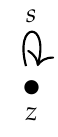and then impose the equation $$s \circ s \circ s \circ s = 1_z$$. You get a category with one object, also known as a monoid. How many morphisms does this category have? How is it related to the picture below?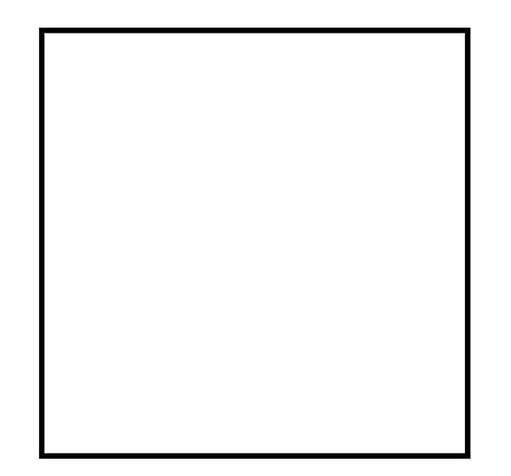Puzzle 108. Take the free category on this graph: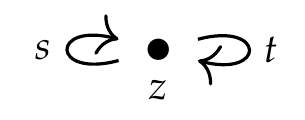and then impose the equations

$$s \circ s \circ s \circ s = t \circ t = s \circ t \circ s \circ t = 1_z .$$ Again you get a monoid. How is it related to the picture below?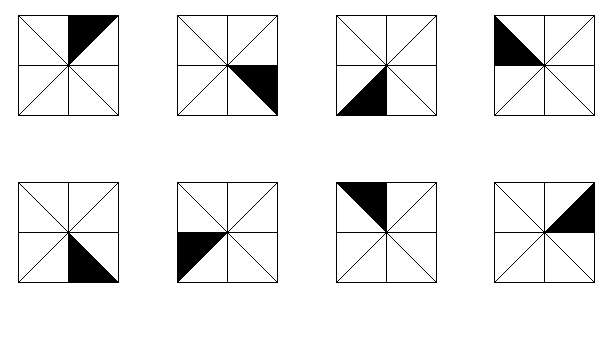These two puzzles are connected to Puzzles 99 and 100. Think about it!

To read other lectures go here.

## Comments

• Options
1.
edited May 2018

I'm excited about these applications of categories to databases!

Comment Source:I'm excited about these applications of categories to databases! 
• Options
2.

I've been fixing a lot of mistakes in this post, so anyone who read it before the time indicated on this comment might want to look at it again now!

Comment Source:I've been fixing a lot of mistakes in this post, so anyone who read it before the time indicated on this comment might want to look at it again now!
• Options
3.
edited May 2018

Puzzle 106. What are some reasonable equations between morphisms that we might want to impose?

Maybe we want to limit the number of layers of management to say 4. $$\text{Manager} \circ \text{Manager} \circ \text{Manager} \circ \text{Manager} = 1_\text{Employee}$$ Puzzle 106-FPE We want to ensure management has a strict hierarchy, i.e. a tree. What equations will enforce that?

Puzzle 107 How many morphisms does this category have? How is it related to the square?

The morphisms are $$\lbrace [1_z = s \circ s \circ s \circ s], [s], [s \circ s], [s \circ s \circ s] \rbrace$$ which are 4.

If $$s$$ represents a $$90 \deg$$ spin of the square then we have a morphism from the initial pose to each symmetric pose of the square [without flipping/turning], of which there are 4.

$$\begin{array}{c|c} \text{Pose} & \text{morphism} \\ \hline \tt{0 \deg} & 1_z = s \circ s \circ s \circ s \\ \tt{90 \deg} & s \\ \tt{180 \deg} & s \circ s \\ \tt{270 \deg} & s \circ s \circ s \end{array}$$

Comment Source:> **Puzzle 106**. What are some reasonable equations between morphisms that we might want to impose? Maybe we want to limit the number of layers of management to say 4. $\text{Manager} \circ \text{Manager} \circ \text{Manager} \circ \text{Manager} = 1_\text{Employee}$ **Puzzle 106-FPE** We want to ensure management has a strict hierarchy, i.e. a tree. What equations will enforce that? > **Puzzle 107** How many morphisms does this category have? How is it related to the square? The morphisms are \$$\lbrace [1_z = s \circ s \circ s \circ s], [s], [s \circ s], [s \circ s \circ s] \rbrace \$$ which are 4. If \$$s\$$ represents a \$$90 \deg\$$ spin of the square then we have a morphism from the initial pose to each symmetric pose of the square [without flipping/turning], of which there are 4. $\begin{array}{c|c} \text{Pose} & \text{morphism} \\\\ \hline \tt{0 \deg} & 1_z = s \circ s \circ s \circ s \\\\ \tt{90 \deg} & s \\\\ \tt{180 \deg} & s \circ s \\\\ \tt{270 \deg} & s \circ s \circ s \end{array}$ 
• Options
4.
edited May 2018

Puzzle 107. Take the free category on this graph:and then impose the equation $$s \circ s \circ s \circ s = 1_z$$. You get a category with one object, also known as a monoid. How many morphisms does this category have? How is it related to the picture below?To simplify the proof, let, $s^{\circ n}=\underbrace{s\circ s\circ s\circ\cdots s}_{n \text{ times}} \\ \#\lbrace s^{\circ n} \rbrace= \#\lbrace f \mid f=s^{\circ n}\rbrace$

When $$n=1$$, $s^{\circ 1}=s \\ \#\lbrace s^{\circ 1} \rbrace= 1.$ When $$n=2$$, $s^{\circ 2}= s\circ s \\ \#\lbrace s^{\circ 2} \rbrace= 1.$

When $$n=3$$, $s^{\circ 3}=s\circ s\circ s \\ \#\lbrace s^{\circ 3} \rbrace= 1.$

When $$n=4$$, $s^{\circ 4}=s\circ s\circ s\circ s=1_z \\ \#\lbrace s^{\circ 4} \rbrace= 0.$ Summing up all the terms, $3=\sum_{n=1}^{4}\#\lbrace s^{\circ n} \rbrace$ Giving an answer of $$3$$.

Edit: Are we also counting $$1_z$$? Otherwise, this gives a different answer.

Comment Source:>**Puzzle 107.** Take the free category on this graph: ><img src = "http://math.ucr.edu/home/baez/mathematical/7_sketches/graph_loop.png"> >and then impose the equation \$$s \circ s \circ s \circ s = 1_z\$$. You get a category with one object, also known as a **[monoid](https://en.wikipedia.org/wiki/Monoid)**. How many morphisms does this category have? How is it related to the picture below? ><img src = "http://math.ucr.edu/home/baez/mathematical/7_sketches/square.png"> To simplify the proof, let, \$s^{\circ n}=\underbrace{s\circ s\circ s\circ\cdots s}\_{n \text{ times}} \\\\ \\#\lbrace s^{\circ n} \rbrace= \\#\lbrace f \mid f=s^{\circ n}\rbrace \$ When \$$n=1\$$, \$s^{\circ 1}=s \\\\ \\#\lbrace s^{\circ 1} \rbrace= 1. \$ When \$$n=2\$$, \$s^{\circ 2}= s\circ s \\\\ \\#\lbrace s^{\circ 2} \rbrace= 1. \$ When \$$n=3\$$, \$s^{\circ 3}=s\circ s\circ s \\\\ \\#\lbrace s^{\circ 3} \rbrace= 1. \$ When \$$n=4\$$, \$s^{\circ 4}=s\circ s\circ s\circ s=1_z \\\\ \\#\lbrace s^{\circ 4} \rbrace= 0. \$ Summing up all the terms, \$3=\sum_{n=1}^{4}\\#\lbrace s^{\circ n} \rbrace \$ Giving an answer of \$$3\$$. Edit: Are we also counting \$$1_z\$$? Otherwise, this gives a different answer. 
• Options
5.

Fredrick Eisele wrote:

Puzzle 106. What are some reasonable equations between morphisms that we might want to impose?

Maybe we want to limit the number of layers of management to say 4. $$\text{Manager} \circ \text{Manager} \circ \text{Manager} \circ \text{Manager} = 1_\text{Employee}$$

This seems to say that each employee is their own manager's manager's manager's manager! That's one way to make sure no management chain is more than four layers deep. Another way might be to say that some top-level employees are their own managers, and that no one is more than four management layers below them.

Puzzle OB1: Try expressing this condition as an equation between two paths.

Comment Source:Fredrick Eisele wrote: >> **Puzzle 106**. What are some reasonable equations between morphisms that we might want to impose? >Maybe we want to limit the number of layers of management to say 4. > $\text{Manager} \circ \text{Manager} \circ \text{Manager} \circ \text{Manager} = 1_\text{Employee}$ This seems to say that each employee is their own manager's manager's manager's manager! That's one way to make sure no management chain is more than four layers deep. Another way might be to say that some top-level employees are their own managers, and that no one is more than four management layers below them. **Puzzle OB1:** Try expressing this condition as an equation between two paths.
• Options
6.
edited May 2018

Presumably the secretary of a department works in that department, so

$$\mathrm{WorksIn} \circ \mathrm{Secretary} = 1_{\mathrm{Department}},$$ though the organisation may have decided otherwise (e.g., put all secretaries in a Secretarial Dept? Or have departments share a secretary - but then the target of WorksIn would have to be the powerset of Departments). Similarly,

$$\mathrm{WorksIn} \circ \mathrm{Manager} = \mathrm{WorksIn}$$ seems reasonable, but not guaranteed.

Comment Source:Presumably the secretary of a department works in that department, so $\mathrm{WorksIn} \circ \mathrm{Secretary} = 1_{\mathrm{Department}},$ though the organisation may have decided otherwise (e.g., put all secretaries in a Secretarial Dept? Or have departments share a secretary - but then the target of WorksIn would have to be the powerset of Departments). Similarly, $\mathrm{WorksIn} \circ \mathrm{Manager} = \mathrm{WorksIn}$ seems reasonable, but not guaranteed.
• Options
7.
edited May 2018

Or have departments share a secretary - but then the target of WorksIn would have to be the powerset of Departments).

Not necessarily. This is where the magic of join tables comes in handy! We could include a new object representing relationships between employees and departments, and have one relation for each department an employee works in.

Puzzle JMC8: What would a presentation of this approach look like?

Comment Source:[Valter wrote](https://forum.azimuthproject.org/discussion/comment/18876/#Comment_18876): > Or have departments share a secretary - but then the target of WorksIn would have to be the powerset of Departments). Not necessarily. This is where the magic of [join tables](https://en.wikipedia.org/wiki/Associative_entity) comes in handy! We could include a new object representing relationships between employees and departments, and have one relation for each department an employee works in. **Puzzle JMC8:** What would a presentation of this approach look like?
• Options
8.

This puzzle is quickly spawning more and more sub-puzzles...

Comment Source:This puzzle is quickly spawning more and more sub-puzzles...
• Options
9.

Valter wrote:

Presumably the secretary of a department works in that department, so

$$\mathrm{WorksIn} \circ \mathrm{Secretary} = 1_{\mathrm{Department}},$$

Yes, this is one of the equations I had in mind!

$$\mathrm{WorksIn} \circ \mathrm{Manager} = \mathrm{WorksIn}$$

Yes, that's the other one! Though as you note, we can imagine exceptions.

Comment Source:Valter wrote: > Presumably the secretary of a department works in that department, so > $\mathrm{WorksIn} \circ \mathrm{Secretary} = 1_{\mathrm{Department}},$ Yes, this is one of the equations I had in mind! > $\mathrm{WorksIn} \circ \mathrm{Manager} = \mathrm{WorksIn}$ Yes, that's the other one! Though as you note, we can imagine exceptions.
• Options
10.
edited May 2018

Keith wrote, regarding Puzzle 107:

Edit: Are we also counting $$1_z$$?

Of course! Identity morphisms are perfectly respectable morphisms just like any other. (Once mathematicians argued about whether 0 was a number, but we decided it was.)

So I think you're saying the answer to Puzzle 107 is "four". That's right, and that's why it has something to do with this picture:Comment Source:Keith wrote, regarding Puzzle 107: > Edit: Are we also counting \$$1_z\$$? Of course! Identity morphisms are perfectly respectable morphisms just like any other. (Once mathematicians argued about whether 0 was a number, but we decided it was.) So I think you're saying the answer to Puzzle 107 is "four". That's right, and that's why it has something to do with this picture: <center><img width = "300" src = "http://math.ucr.edu/home/baez/mathematical/7_sketches/square.png"></center>
• Options
11.
edited June 2018

As it stands, such a group $$(\mathcal{G},\circ )$$, with $$g^{\circ n}=1_\mathcal{G}$$ is a cyclic group.

So the above is a cyclic group on 4 elements.

Comment Source:As it stands, such a group \$$(\mathcal{G},\circ )\$$, with \$$g^{\circ n}=1_\mathcal{G}\$$ is a [cyclic group](https://en.wikipedia.org/wiki/Cyclic_group). So the above is a cyclic group on 4 elements.
• Options
12.

Puzzles 107 and 108: ooh, these are fun. OK, so there seems to be a connection between finitely presented categories and symmetry groups. For 107, we can interpret s as a 90 degree rotation. For 108, we can do the same for s, and have t be either an x-axis reflection or a y-axis reflection.

RCG2: for 108, I gave two interpretations of t. Are there more? What about different interpretations of s? Are all interpretations unique up to isomorphism?

Questions: is the notion of "interpretation" I'm informally using formalizable as a functor? For instance, let F be the map from the category in 107 to the 90 rotation group of a square (maybe let's say that the square has a marked orientation, like a red dot on the top right corner). If F maps from the one object of the category to the one object of the group (viewed as a category), then F is a functor. (Digression: ooh, this can't be quite the right notion of interpretation, because I want to distinguish the interpretation of t as an x-axis flip from the interpretation of t as a y-axis flip, but presumably the symmetry groups are isomorphic? I don't know any group theory, so this is all a bit speculative).

If so, then I could ask RCG3: is it the case that for any finitely presentable one object category C, there exist a functor F from C to a symmetry group? And if there are two such functors F and G, is F(C) isomorphic to G(C)?

I might be getting a bit ahead of myself - not yet sure this is a well-formed question.

Comment Source:Puzzles 107 and 108: ooh, these are fun. OK, so there seems to be a connection between finitely presented categories and symmetry groups. For 107, we can interpret s as a 90 degree rotation. For 108, we can do the same for s, and have t be either an x-axis reflection or a y-axis reflection. RCG2: for 108, I gave two interpretations of t. Are there more? What about different interpretations of s? Are all interpretations unique up to isomorphism? Questions: is the notion of "interpretation" I'm informally using formalizable as a functor? For instance, let F be the map from the category in 107 to the 90 rotation group of a square (maybe let's say that the square has a marked orientation, like a red dot on the top right corner). If F maps from the one object of the category to the one object of the group (viewed as a category), then F is a functor. (Digression: ooh, this can't be quite the right notion of interpretation, because I want to distinguish the interpretation of t as an x-axis flip from the interpretation of t as a y-axis flip, but presumably the symmetry groups are isomorphic? I don't know any group theory, so this is all a bit speculative). If so, then I could ask RCG3: is it the case that for any finitely presentable one object category C, there exist a functor F from C to a symmetry group? And if there are two such functors F and G, is F(C) isomorphic to G(C)? I might be getting a bit ahead of myself - not yet sure this is a well-formed question. 
• Options
13.
edited June 2018

Nice answers, Reuben!

Questions: is the notion of "interpretation" I'm informally using formalizable as a functor?

Yes! If you have a category $$\mathcal{C}$$ with one object and with all morphisms invertible, it's secretly just a group. And if you have a functor $$F: \mathcal{C} \to \mathbf{Set}$$, it gives a set and an action of your group as permutations of that set.

For example, your set could be the 4 corners of the square, or the set of all points in the square. Your category $$\mathcal{C}$$ could be the one in Puzzle 107. The functor $$F : \mathcal{C} \to \mathbf{Set}$$ sends the one object $$z$$ in $$\mathcal{C}$$ to your set. It sends the four morphism $$1_z, s, s^2, s^3$$ in this category to the four rotations of your set!

More generally, for any $$\mathcal{C}$$ , a functor $$F: \mathcal{C} \to \mathbf{Set}$$ gives you a bunch of sets (one for each object in your category) and functions (one for each morphism) obeying the same relations as the morphisms in your category. So, it gives a "concrete picture" of the abstract category $$\mathcal{C}$$.

I think this all I will say now, but there's a lot more to say here.

Comment Source:Nice answers, Reuben! > Questions: is the notion of "interpretation" I'm informally using formalizable as a functor? Yes! If you have a category \$$\mathcal{C}\$$ with one object and with all morphisms invertible, it's secretly just a group. And if you have a functor \$$F: \mathcal{C} \to \mathbf{Set}\$$, it gives a set and an action of your group as permutations of that set. For example, your set could be the 4 corners of the square, or the set of all points in the square. Your category \$$\mathcal{C}\$$ could be the one in Puzzle 107. The functor \$$F : \mathcal{C} \to \mathbf{Set}\$$ sends the one object \$$z\$$ in \$$\mathcal{C}\$$ to your set. It sends the four morphism \$$1_z, s, s^2, s^3\$$ in this category to the four rotations of your set! More generally, for any \$$\mathcal{C}\$$ , a functor \$$F: \mathcal{C} \to \mathbf{Set}\$$ gives you a bunch of sets (one for each object in your category) and functions (one for each morphism) obeying the same relations as the morphisms in your category. So, it gives a "concrete picture" of the abstract category \$$\mathcal{C}\$$. I think this all I will say now, but there's a lot more to say here.
• Options
14.

Given our one-object category, does the functor $$F$$ always produce a square out of the elements of the chosen set?

What happens if we change base to $$\mathbf{Cost}$$? Do we get a square with sides that have a specific length?

Such squares and other simple geometric shapes and their properties were studied by the ancient Greeks extensively. Can the mathematics of the ancient Greeks be defined as a functor or $$\mathcal{V}$$-functor?

Comment Source:Given our one-object category, does the functor \$$F\$$ always produce a square out of the elements of the chosen set? What happens if we change base to \$$\mathbf{Cost}\$$? Do we get a square with sides that have a specific length? Such squares and other simple geometric shapes and their properties were studied by the ancient Greeks extensively. Can the mathematics of the ancient Greeks be defined as a functor or \$$\mathcal{V}\$$-functor?
• Options
15.
edited June 2018

RCG3:

The morphisms of a category $$\mathscr{C}$$ presented by graph ($$\mathscr{G} = (E,{\bullet})$$) with only one node, and equations ($$Eq \subset E^\x E^$$ where $$Eq = [\forall x y, x\circ y = y \circ x] \cup Eq' where (\forall(x = y)\in Eq', y = id,\,\land\,\forall e\in E, \exists x, e \in x \land (x = id) \in Eq'$$ are an albien group under composition.

Proof: We know that all one object categories are monoids (and vice versa). So we just have to show that each element has an unique inverse. By the definition of presented in: $\forall m \in \mathscr{Hom}(\bullet,\bullet), \exists w \in E^* of length n, m = w_0\circ w_1 \circ \dots \circ w_{n-1}$ in fact there are many such w because of the equations in Eq.

Proceed by Induction: $w=\epsilon,$ in which case m = id = id^{-1}\] $\exists m' m'^{-1}, \m = w_0\circ m'\ \land m'\circ m'_{-1}=id) If w_0 has an inverse then: \[w_0 m'\circ [m'^{-1}w_0^{-1}] = m\circ m'^{-1}w_0^{-1} =id = [m'^{-1}w_0^{-1}] \circ m$

So we just need to find an inverse to the singleton word [w_0]. By assumption, $\exists x, w_0 \in x \land (x,id) \in Eq'$ by the $$\forall x y, x\circ y = y\circ x$$ equation we can pull an w_0 to the front (using $$\backslash$$ as list difference, not set difference): $w_0 (x \backslash w_0) = id$ so $$w_0^{-1} = x \backslash w_0$$.

And we have what we need.

I am pretty sure its not that hard to extend this too the non ablian case. But cases like "fgfgh=id" give me headaches.

I also have no idea how to tell if a group is an symetry group.

Comment Source:RCG3: The morphisms of a category \$$\mathscr{C}\$$ presented by graph (\$$\mathscr{G} = (E,\{\bullet\})\$$) with only one node, and equations (\$$Eq \subset E^*\x E^*\$$ where \$$Eq = [\forall x y, x\circ y = y \circ x] \cup Eq' where (\forall(x = y)\in Eq', y = id,\,\land\,\forall e\in E, \exists x, e \in x \land (x = id) \in Eq'\$$ are an albien group under composition. Proof: We know that all one object categories are monoids (and vice versa). So we just have to show that each element has an unique inverse. By the definition of presented in: \$\forall m \in \mathscr{Hom}(\bullet,\bullet), \exists w \in E^* of length n, m = w_0\circ w_1 \circ \dots \circ w_{n-1}\$ in fact there are many such w because of the equations in Eq. Proceed by Induction: \$w=\epsilon,\$ in which case m = id = id^{-1}\\] \$\exists m' m'^{-1}, \m = w_0\circ m'\\ \land m'\circ m'_{-1}=id) If w_0 has an inverse then: \\[w_0 m'\circ [m'^{-1}w_0^{-1}] = m\circ m'^{-1}w_0^{-1} =id = [m'^{-1}w_0^{-1}] \circ m\$ So we just need to find an inverse to the singleton word [w_0]. By assumption, \$\exists x, w_0 \in x \land (x,id) \in Eq'\$ by the \$$\forall x y, x\circ y = y\circ x\$$ equation we can pull an w_0 to the front (using \$$\backslash\$$ as list difference, _not_ set difference): \$w_0 (x \backslash w_0) = id\$ so \$$w_0^{-1} = x \backslash w_0\$$. And we have what we need. I am pretty sure its not that hard to extend this too the non ablian case. But cases like "fgfgh=id" give me headaches. I also have no idea how to tell if a group is an symetry group. 
• Options
16.

An open question to everyone: How would a person get across the constraint that in (a standard) firm, every employee shares a unique employer, the employer of the firm (which may be a single person or set of people)?

That is to say, every employee is hired by the employer, who then commands their labor, either directly or through a chain of special employees called managers.

Furthermore, could we also add the constraint that the employer, being the contractual nexus of hiring, renting, or already owning, all the inputs used up in production (and thus the legal party who "swallowed" those liabilities), is also the legal party who ends up appropriating (i.e., having the defensible claim on) the produced assets (as is the case in almost all countries nowadays)?

Comment Source:An open question to everyone: How would a person get across the constraint that in (a standard) firm, every employee shares a unique employer, *the employer of the firm* (which may be a single person or set of people)? That is to say, every employee is *hired* by the employer, who then commands their labor, either directly or through a chain of special employees called *managers*. Furthermore, could we also add the constraint that the employer, being the contractual nexus of hiring, renting, or already owning, all the inputs used up in production (and thus the legal party who "swallowed" those liabilities), is also the legal party who ends up appropriating (i.e., having the defensible claim on) the produced assets (as is the case in almost all countries nowadays)?
• Options
17.

Keith #16 - given our approach so far, we cannot impose a constraint saying that everyone has the same employer.

"Our approach so far" is this: we specify any category $$\mathcal{C}$$ whatsoever as our database schema. Then, a database is a functor $$F: \mathcal{C} \to \mathbf{Set}$$.

In this approach, if we have two databases

$$F: \mathcal{C} \to \mathbf{Set}$$ $$G: \mathcal{C} \to \mathbf{Set}$$ we can always form their sum, or coproduct, or intuitively 'disjoint union'

$$F + G: \mathcal{C} \to \mathbf{Set}$$ which on objects has

$$(F + G)(x) := F(x) + G(x)$$ where at right $$F(x) + G(x)$$ is the disjoint union of the sets $$F(x)$$ and $$G(x)$$.

So, if $$\mathcal{C}$$ has a morphism

$$\textrm{EmployerOf} : \textrm{Employee} \to \textrm{Person}$$ any database $$F$$ will say that each employee has one person who is their employee, thanks to the function

$$F(\textrm{EmployerOf}): F(\textrm{Employee}) \to F(\textrm{Person}).$$ However, we cannot impose the constraint that everyone has the same employee, because we can slap two databases together and get

$$(F+G)(\textrm{EmployerOf}): F(\textrm{Employee}) + G(\textrm{Employee}) \to F(\textrm{Person}) + G(F(\textrm{Person}).$$ There are fancier theories of databases, where we can do more things! But we're starting with the simplest, which has certain limitations.

Comment Source:Keith #16 - given our approach so far, we cannot impose a constraint saying that everyone has the same employer. "Our approach so far" is this: we specify any category \$$\mathcal{C}\$$ whatsoever as our database schema. Then, a database is a functor \$$F: \mathcal{C} \to \mathbf{Set}\$$. In this approach, if we have two databases $F: \mathcal{C} \to \mathbf{Set}$ $G: \mathcal{C} \to \mathbf{Set}$ we can always form their sum, or coproduct, or intuitively 'disjoint union' $F + G: \mathcal{C} \to \mathbf{Set}$ which on objects has $(F + G)(x) := F(x) + G(x)$ where at right \$$F(x) + G(x)\$$ is the disjoint union of the sets \$$F(x)\$$ and \$$G(x)\$$. So, if \$$\mathcal{C}\$$ has a morphism $\textrm{EmployerOf} : \textrm{Employee} \to \textrm{Person}$ any database \$$F\$$ will say that each employee has one person who is their employee, thanks to the function $F(\textrm{EmployerOf}): F(\textrm{Employee}) \to F(\textrm{Person}).$ However, we cannot impose the constraint that _everyone has the same employee_, because we can slap two databases together and get $(F+G)(\textrm{EmployerOf}): F(\textrm{Employee}) + G(\textrm{Employee}) \to F(\textrm{Person}) + G(F(\textrm{Person}).$ There are fancier theories of databases, where we can do more things! But we're starting with the simplest, which has certain limitations.
Sign In or Register to comment.